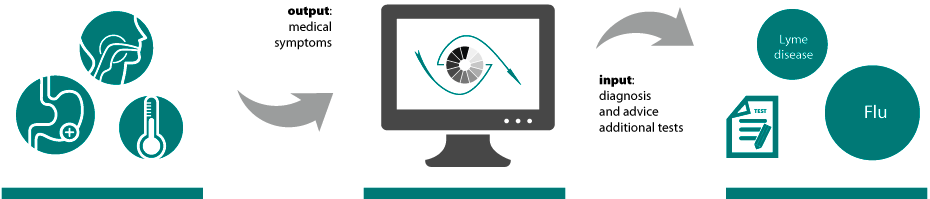# What is Machine Learning?

Machine Learning is the scientific discipline that deals with the development of algorithms that allow computers to learn and reason. These algorithms are on the basis of data, domain knowledge, or a combination of both. In the field of Machine Learning, there are several possibilities. The type model depends on the type of problem, the available data and existing domain knowledge. We roughly distinguish the following two types of problems: prediction and reasoning problems.

## Prediction problems

Prediction problems are of the form "given the input, what is the output?". When we talk about a prediction problem, we must predict an output based on input. The output is predicted on the basis of a model which built based on historical inputs and outputs. To illustrate an example of a prediction problem: predicting stock prices. (from here read more button). We possess data from the past (input), but we cannot make any statements without mathematical models on tomorrows stock prices (output). The input exists in this case of stock prices combined with weather data, traffic data and news events of the past few years.

By analyzing this data, patterns can be detected. For example: the weather and traffic congestion affects the prices of stocks. The discovered relationships between the variables form the basis of the mathematical model that Big4Data develops. This mathematical model is then translated into a practical application which can predict the stock prices with a greater degree of certainty. When we talk about a prediction problem we are trying to detect the underlying mechanisms of a particular phenomenon. This knowledge can then be used to make better predictions in the future. Stock market pricing is not the only example of a prediction problem. Other examples include prediction of consumer behavior, or the prediction of natural phenomena.## Reasoning Problems

Reasoning Problems are problems of the form: Given the output, what are the possible inputs'. In reasoning problems, the output is evident and the cause for the output must be determined from data. We illustrate the reasoning problem by using the example of medical diagnosis. The input for the model are data that have been collected in the past (symptoms combined with diagnoses) and domain knowledge. There are connections between certain symptoms and diagnoses. These are incorporated in a model that is then translated into a practical application. In the case of a reasoning problem the output known; the patient has a fever, sore throat and stomach upset. The doctor will perform some tests and enter the results of the tests and the symptoms in the program.

There are connections between certain symptoms and diagnoses. These are incorporated in a model that is then translated into a practical application. In the case of a reasoning problem the output known; the patient has a fever, sore throat and stomach upset. The doctor will perform some tests and enter the results of the tests and the symptoms in the program. Next, the program reasons to a diagnosis. The system is particularly suitable to show possible alternatives that a physician might overlook. A doctor may conclude based on the given symptoms that the patient has the flu. The system then shows that there is also a possibility that the patient has been bitten by a tick, because these symptoms can also indicate Lyme disease. Furthermore, the reasoning system can suggest additional tests that can further support or reject the diagnosis. Reasoning Problems exist not only in the case of medical diagnostics. Other examples include the system that Big4Data developed for Shell and for the NFI.### Bayesian Networks

A lot of reasoning models that Big4Data builds, are based on Bayesian network technology. A Bayesian network is a data structure that is used to model probability distributions. Such a network can be seen as a diagram in which events and the relationships between them are described. (From here read more button) Bayesian networks are models in which probability theory is applied in order to deal with uncertainty. This uncertainty is usually caused by missing information or incomplete knowledge.

The degree of certainty / uncertainty is explained by the example of a medical diagnosis (the example that was used earlier when discussing the reasoning problem). There are thousands of tests to diagnose, but it is impossible to subject a patient to thousands of medical tests. The diagnosis is made on the basis of the output of the most obvious tests. The degree of uncertainty caused by the absence of the results of the other tests, poses no problems for probability based approach.